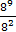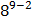### Sample Problem

Simplify the following expression. Leave your answer in the form a^b=

#### Solution

Quotient of Powers Property

when you divide two powers with the same base, you subtract the exponents. In other words, for all real numbers ab, and c, where a ≠ 0,So==